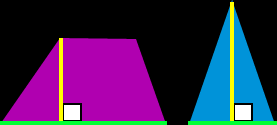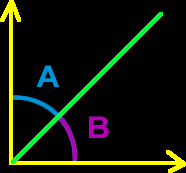# Online Math Dictionary: B

Easy to understand math definitions for K-Algebra mathematics
Just scroll down or click on the word you want and I'll scroll down for you!

 base biannual binary binomial bisect bisector

 BaseIn geometry, the base of a shape is the side (usually the bottom) that forms a right (90 degree) angle with the height of the object.Biannual
Biannual means twice a year.  It's the same as semi-annual.  The term shows up in a lot of money problems in algebra.
Example:  If interest is compounded biannually, then it's compounded twice a year.Binary
Our regular number system is "base 10" which means that we use TEN digits: 0, 1, 2, 3, 4, 5, 6, 7, 8, 9.  But, there are other number systems.  The binary number system is "base 2" which means that it uses just two digits: 0 and 1.   Computers speak in binary numbers!
Example:  110101 is binary for 53 in our regular number system (base 10).Binomial
A binomial is a polynomial with two terms...  Example:   5x + 6y
 Bisect(Verb) To bisect something (like an angle) is to cut it in half.   In the pic on the rightBisector(Noun) A bisector is the line that cuts  something (like an angle) in half.